# NCERT Solutions Class 12 Economics Chapter-12 (Market Equilibrium with Simple Applications)

NCERT Solutions Class 12 Economics from class 12th Students will get the answers of Chapter-12 (Market Equilibrium with Simple Applications) This chapter will help you to learn the basics and you should expect at least one question in your exam from this chapter.
We have given the answers of all the questions of NCERT Board Economics Textbook in very easy language, which will be very easy for the students to understand and remember so that you can pass with good marks in your examination.

Class 12 Economics

Chapter-12 (Market Equilibrium with Simple Applications)

Questions and answers given in practice

Chapter-12 (Market Equilibrium with Simple Applications)

Question 1. Explain market equilibrium. [1 Mark]
Answer: Market equilibrium refers to the situation when market demand is equal to the market supply.

Question 2. What will happen if the price prevailing in the market is
(i) Above the equilibrium price?
(ii) Below the equilibrium price?
[6 Marks] Or
How price and quantity are determined in the market when number of firms are fixed? Or
How is equilibrium price of a commodity determined? (Use diagram).
[CBSE 2004C; AI 07, 09] Or
Explain why equilibrium price is determined at the level of output at which its demand is equal to its supply. [CBSE 2010C]
Or
How will equilibrium price be reached when there is excess demand/excess supply? Explain with diagram. [CBSE 2004, 07; AI 2004] Or
With the help of a suitable diagram, explain the process of determination of equilibrium price of a commodity under perfectly competitive market.
[CBSE Sample Paper 2003] Or
Market for a good is in equilibrium. Explain the chain of reactions in the market if the price is
(i) higher than equilibrium price and
(ii) lower than equilibrium price. [AI 2012]

1. Market equilibrium refers to that point which has come to be established under a given condition of demand and supply and has a tendency to stick to that level, i.e. where Demand = Supply.
2. If due to some disturbance we divert from our position the economic forces will work in such a manner that it could be driven back to its original position, i.e., where Demand = Supply. In short it is the position of rest.
3. It can be explained with the help of following schedule and diagram:
(a) • In the below schedule market equilibrium is determined at Price 3 where Market demand is equal to Market Supply.
• At price 1 and 2, there is excess demand, which leads to rise in price, resulting tendency is expansion in supply.
• Similarly, at price 4 and 5, there is excess supply, which leads to fall in price, resulting tendency is Contraction in supply.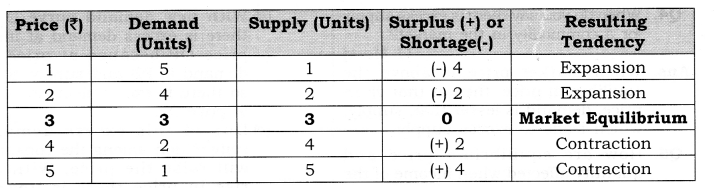(b) • In the given diagram, price is measured on vertical axis, whereas quantity demanded and supply is measured on horizontal axis.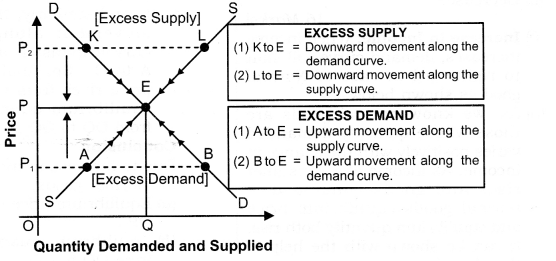• Suppose that initially the price in the market is P1. At this price, the consumer demand P1B and the producer supply P1A, i.e. consumers want more than what the producer are willing to supply. There is excess demand equal to AB. So, price cannot stay on P1 as excess demand will create competition among the buyers and push the price up till we reach equilibrium. Due to rise in price from P1 to P, there is upward movement along the supply curve (expansion in supply) from A to E and upward movement along the demand curve (contraction in demand) from B to E.
• Similarly, at price P2, the quantity demanded P2K is less than the quantity supplied P2L. There is excess supply, equal to KL, which will create competition among the sellers and lower the price. The price will keep falling as long as there is an excess supply.
Due to fall in price from P2 to P there is downward movement along the supply curve (contraction in supply) from L to E and downward movement along the demand curve (expansion in demand) from K to E.
• The situation of zero excess demand and zero excess supply defines market equilibrium (E). Alternatively, it is defined by the equality between quantity demanded and quantity supplied. The price P is called equilibrium price and quantity Q is called equilibrium quantity.

Question 3. When do we say there is excess demand for a commodity in the market? [1 Mark]
Answer: When Market price is below the equilibrium price, then at that given price, demand is greater than supply, which leads to excess demand.

Question 4. When do you say there is excess supply for a commodity in the market? [1 Mark]
Answer: When Market price is above the equilibrium price, then at that given price, demand is lesser than supply, which leads to excess supply.

Question 5. How are equilibrium price and quantity affected when income of the consumers

1.  Increase?
2. Decrease?
[6 Marks]

1. Increase in Income: When income increases, demand curve will shift to rightward in case of Normal good as shown below:
(a) As, we know normal goods are those whose quantity demanded varies positively with the change in income. As income of a consumer rises and goods consumed is normal goods equilibrium price and equilibrium quantity both rise. It can be shown with the help of the given figure.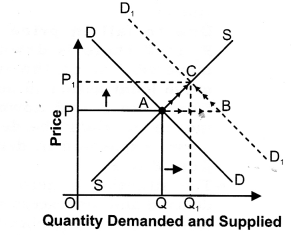(b) In the given figure, price of normal goods is measured on vertical axis and quantity demanded and supplied are measured on horizontal axis. Initially, the equilibrium price is OP and equilibrium quantity is OQ.
(c) But as given in the examination problem when income of a consumer rises the demand of normal goods increases shifting the demand curve to the right from DD to D1D1.
(d) With new demand curve DJDJ, there is excess demand at initial price OP because at price OP demand is PB and supply is PA; so there is excess demand of AB at price OP.
(e) Due to this excess demand, competition among the consumer will raise the price. With the rise in price there is upward movement along the demand curve (contraction in demand) from B to C and similarly, there is upward movement along the supply curve (expansion in supply) from A to C . So, finally, equilibrium price rises from OP to OPi; and equilibrium quantity also rises from OQ to OQr
Conclusion
Due to increase in income of a buyer
for normal goods,
(a) Equilibrium price rises from OP to OP1.
(b) Equilibrium quantity also rises from OQ to OQ1.
2. Decrease in income: When income decreases, demand curve will shift to leftward in case of Normal good as shown below:
(a) As we know that normal goods are those whose quantity demanded varies positively with the change in income. As given in the examination problem if income of a consumer falls and goods consumed is normal goods, then both equilibrium price and the equilibrium quantity fall. It can be shown with the help of the given figure.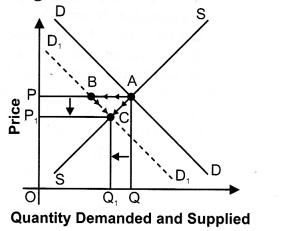(b) In the given figure price of normal goods is measured on vertical axis and quantity demanded and supplied is measured on horizontal axis. Initially, the equilibrium price is OP and equilibrium quantity is OQ.
(c) But as given in the examination problem when income of a consumer falls the demand of normal goods also falls shifting the demand curve to the left from
DD to D1D1.
(d) With new demand curve D1D1 there is excess supply at initial price OP because at price OP demand is PB and supply is PA; so there is excess supply of AB at price OP.
(e) Due to this excess supply competition among the producer will fall the price. Due to fall in price there is downward movement along the demand curve (Expansion in demand) from B to C and similarly, there is downward movement along the supply curve (Contraction in supply) from A to C. So, finally, the equilibrium price falls from OP to OP1 and equilibrium quantity also falls from OQ to OQ1

Conclusion
Due to decrease in income of a buyer for normal goods,

1. Equilibrium price falls from OP to OP1.
2. Equilibrium quantity also falls from OQ to OQ1.

Question 6. Using supply and demand curves, show how an increase in the price of shoes affects the price of a pair of socks and the number of pairs of socks bought and sold.
Or
How will a rise in price of complementary affect the equilibrium price of given commodity? Explain the chain of effects. [6 Marks]

1. As we know, shoes and pair of socks are complementary good to each other. As, price of complementary goods are inversely related with the demand of given commodity. So, rise in price of shoes (complementary good) decreases the demand for given commodity (pair of socks), and demand curve shifts leftward as shown in given figure: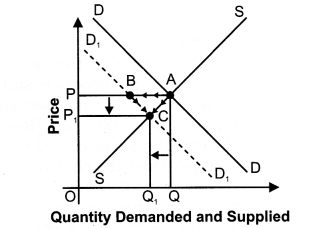2.  In the given diagram, price is on vertical axis and quantity demanded and supplied is on horizontal axis. Initially, the equilibrium price is OP and equilibrium quantity is OQ.
3. But due to rise in price of complementary good the demand curve of given commodity shifts leftward from DD to D1D1.
4. With new demand curve D1D1 there is excess supply at initial price OP because at price OP demand is PB and supply is PA; so, there is excess supply of AB at price OP.
5. Due to this excess supply, competition among the producer will fall the price. Due to fall in price, there is downward movement along the demand curve (Expansion in demand) from B to C and similarly there is downward movement along the supply curve (Contraction in supply) from A to C. So, finally, the equilibrium price falls from OP to OP1 and equilibrium quantity also falls from OQ to OQ1 So, due to rise in price of complementary goods,
(a) Equilibrium price falls from OP to OP1 and
(b) Equilibrium quantity also falls from OQ to OQ1
MORE QUESTIONS SOLVED

I.Very Short Answer Type Questions (1 Mark)
Question 1. Define market equilibrium. [CBSE Foreign 2011]
Answer: Market equilibrium refers to the situation when market demand is equal to the market supply.

Question 2. Give the meaning of equilibrium price. [CBSE, Sample Paper 2010]
Answer: The price at which equilibrium is reached is called equilibrium price.

Question 3. Give the meaning of equilibrium quantity.
Answer: The quantity bought and sold at the equilibrium price is called equilibrium quantity.

Question 4. What is equilibrium point?
Answer: Equilibrium point is the point of intersection of the demand curve and supply of commodity.

Question 5. When do you say there is excess demand for a commodity in the market?
Answer: When Market price is below the equilibrium price, then at that given price, demand is greater than supply that leads to excess demand.

Question 6. When do you say there is excess supply for a commodity in the market?
Answer: When market price is above the equilibrium price, then at that given price, demand is lesser than supply, that leads to excess supply.

Question 7. For a non-viable industry where does the supply curve lie relative to demand curve?
Answer: Supply curve lies above the demand curve.

Question 8. A severe drought results in a drastic fall in the output of wheat. Analyse how will it affect the market price of wheat?
Answer: Market price of wheat will increase (due to decrease in supply).

Question 9. What happens to equilibrium price of a commodity if there is ‘decrease’ in its demand and ‘increase’ in its supply? [CBSE Sample Paper 2008]

Question 10. What happens to equilibrium price of a commodity if there is an ‘increase’ in its demand and ‘decrease’ in its supply? [CBSE Sample Paper 2010]

II. Multiple Choice Questions (1 Mark)
Question 1. If price is above then equilibrium Price, there is:
(a) excess demand
(b) excess supply
(c) price ceiling
(d) price flooring

Question 2. With a given supply curve a decrease in demand causes—————-.
(a) an overall decrease in price but an increase in equilibrium quantity.
(b) an overall increase in price but a decrease in equilibrium quantity.
(c) an overall decrease in price and a decrease in equilibrium quantity.
(d) no change in overall price but a reduction in equilibrium quantity.

Question 3. Assume that consumers’ incomes and the number of sellers in the market for goods A both decrease. Based upon this information, we can conclude, with certainty, that the equilibrium
(a) price will increase.
(b) price will decrease.
(c) quantity will increase.
(d) quantity will decrease.

Question 4. Suppose that the supply of cameras increases due to an increase in imports. Which of the following statements will most likely occur?
(a) The equilibrium price of cameras will increase.
(b) The equilibrium quantity of cameras exchanged will decrease.
(c) The equilibrium price of camera film will decrease.
(d) The equilibrium quantity of camera film exchanged will increase.

Question 5. Assume that in the market for a good Z there is a simultaneous increase in demand and the quantity supplied. The result will be:
(a) An increase in equilibrium price and quantity.
(b) A decrease in equilibrium price and quantity.
(c) An increase in equilibrium quantity and uncertain effect on equilibrium price.
(d) A decrease in equilibrium price and increase in equilibrium quantity.

Question 6. Suppose the technology for producing personal computers improves and, at the same time, individuals discover new uses for personal computers so that there is greater utilization of personal computers. Which of the following statements/factors will happen to equilibrium price and equilibrium quantity?
(a) Price will increase; quantity cannot be determined.
(b) Price will decrease; quantity cannot be determined.
(c) Quantity will increase; price cannot be determined.
(d) Quantity will decrease; price cannot be determined.

Question 7. When there is increase in demand and decrease in supply, equilibrium price:
(a) Falls (b) Rises
(c) Constant (d) None of these

III. Short Answer Type Questions  (3-4 Marks)
Question 1. Under what condition increase in demand would not make any effect on equilibrium price?
Answer: Case I: When supply also increase at the same rate as the demand increases
In the given diagram price is measured on vertical axis and quantity demanded and supplied is measured on horizontal axis. Initially, the equilibrium price is OP and equilibrium quantity is OQ. But when “demand and supply both increase at the same rate” then,

Case II: When supply becomes perfectly elastic – In the given diagram price is measured on vertical axis and quantity demanded and supplied is measured on horizontal axis. Initially, the equilibrium price is OP and equilibrium quantity is OQ. But when “supply becomes perfectly elastic and demand increases then,

Question 2. Under what condition increase in demand would not make any effect on equilibrium quantity?
Answer: Case I: When supply decreases at the same rate as the demand increase
In the given diagram price is measured on vertical axis and quantity demanded and supplied is measured on horizontal axis. Initially, the equilibrium price is OP and equilibrium quantity is OQ.
But when, “demand increases and supply decreases but at the same rate”, then,

Case II: When supply becomes perfectly inelastic
In the given diagram price is measured on vertical axis and quantity demanded and supplied is measured on horizontal axis. Initially, the equilibrium price is OP and equilibrium quantity is OQ.
But when “supply becomes perfectly inelastic and demand increase” then,

Question 3. Give reasons for the following statements:

1. A decrease in supply will not result in a change in equilibrium quantity if the demand for a commodity is perfectly inelastic.
2. An decrease in supply will not result in a change in equilibrium price if the demand for a commodity is perfectly elastic.

1.  If the demand for a commodity is perfectly inelastic, i.e., if the demand curve is a vertical straight line, a decrease in supply curve will result only in a change in the equilibrium price, but no change in the equilibrium quantity.
2. If the demand for a commodity is perfectly elastic, i.e., if the demand curve is a horizontal straight line, a decrease in supply curve will result only in change equilibrium quantity, but no change in equilibrium price.

Question 4. Explain the effects of a ‘price ceiling’. [CBSE Sample Paper 2014] Or
Explain the effects of ‘maximum price ceiling’ on the market of a good. Use diagram. [CBSE 2015]

1. When the government imposed upper limit on the price (maximum price) of a good or service which is lower than equilibrium price is called price ceiling.
2. Price ceiling is generally imposed on necessary items like wheat, rice, kerosene etc.
3. It can be explained with the help of diagram below: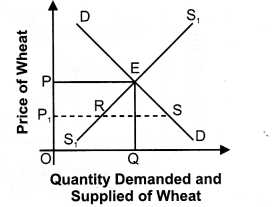(a) In the given diagram, DD is the market demand curve and SS is the market supply curve of Wheat. Suppose, equilibrium price OP is very high for many individuals and they are unable to afford at this price.
(b) As wheat is necessary product, government has to intervene and impose price ceiling of Pi; which is below the equilibrium level.
(c) Since this price is below equilibrium price, there is excess demand in the market. With shortages, sellers tend to hoard the product. It could also lead to black marketing.

Question 5. Explain the effects of a ‘price floor’. [CBSE Sample Paper 2014] Or
What are the effects of ‘price – floor’ (minimum price ceiling) on the market of a good? Use diagram.

1. When the government imposed lower limit on the price (minimum price) that may be charged for a good or service which is higher than equilibrium price is called price floor.
2. Price Floor is generally imposed on agricultural price support programmes and the minimum wage legislation.
3. Since this price is above equilibrium price, there is excess supply in the market. Since there is surplus, sellers can attempt to sell their product at a price below the floor price.IV. True Or False
Giving reasons, state whether the following statements are true or false:
Question 1. Equilibrium between demand and supply helps in determining prevailing price of the product.
Answer: False: An equilibrium between demand and supply determines only equilibrium price.

Question 2. In case of excess demand, equilibrium price is less than prevailing price.
Answer: False: When there is excess demand, equilibrium price is greater than prevailing price.

Question 3. In case of excess supply, equilibrium price is greater than prevailing price.
Answer: False: When there is excess supply, equilibrium price is less than prevailing price.

Question 4. When supply decreases more than proportionately than the fall in demand, equilibrium price will also fall.
Answer:  False: With a decrease in supply proportionately more than demand, equilibrium price will rise.

Question 5. When demand increases more than proportionately than the increase in supply, equilibrium price will fall.
Answer: False: When demand increases proportionately more than increase in supply, equilibrium price will rise.

Question 6. When income of buyers increase, equilibrium price falls.
Answer: False: With an increase in income of buyers, equilibrium price will increase when piece of goods is normal, but decreases when that is inferior.

Question 7. In a state of equilibrium, quantity demanded will be less than the quantity supplied.
Answer: False: Market equilibrium is obtained when the quantity supplied of a commodity equals the quantity demanded. If the quantity demanded of a commodity is less than the quantity supplied, equilibrium price will fall.

Question 8. If the demand for a commodity increases, its supply curve remaining the same, the market price of the commodity will rise.
Answer: True: Increase in demand implies a rightward shift of the demand curve. New demand curve will intersect the given supply curve at a higher price. The equilibrium price will rise.

Question 9. An increase in supply results in a fall both in equilibrium quantity and equilibrium price.
Answer: False: Increase in supply implies a rightward shift of the supply curve. New supply curve will intersect the given demand curve at a lower price. The equilibrium price will fall; equilibrium quantity will rise.

Question 10. If the increase in demand is proportionately equal to the decrease in supply, equilibrium price will rise.
Answer: True: As we know whether demand increases or supply decreases both lead to an increase in equilibrium price. Hence, equilibrium price will rise.

Question 11. If the decrease in demand meets with an increase in supply, equilibrium price will fall.
Answer: True: As we know whether demand decreases or supply increases both lead to an decrease in equilibrium price. Hence, equilibrium price will fall.

Question 12. Equilibrium price will not change if the decrease in demand meets with a proportionate decrease in supply.
Answer: True: Decrease in demand results in a fall in equilibrium price; decrease in supply, on the other hand, results in an increase in price. If both the changes are proportionately equal, equilibrium price will not change.

Question 13. If the supply curve is a vertical straight line, change in demand will not affect equilibrium price.
Answer:False: A vertical straight line supply curve implies that the quantity supplied cannot be changed. If the demand increases, equilibrium price will increase proportionately.

Question 14. If the supply curve is a horizontal straight line, change in demand will affect equilibrium quantity.
Answer: True: A horizontal straight line supply curve implies that the quantity supplied can be adjusted to the change in demand. With the increase in demand, with no corresponding increase in price, equilibrium quantity will increase.
Note: As per CBSE guidelines, no marks will be given if reason to the answer is not explained.

V. Long Answer Type Questions  (6 Marks)
Question 1. If at a given price of the commodity there is excess demand, how will the equilibrium price be reached? Explain with the help of a diagram.
Or
[CBSE 2004] If equilibrium price of a good is greater than its market price, explain all the changes that will take place in the market. Use diagram.
[AI 2013] Or
Explain the changes that will take place in the market for a commodity if the prevailing market price is less than the equilibrium price. [CBSE Sample Paper 2011]

Answer: If at a given price let at P1( there is excess demand as shown in the given figure.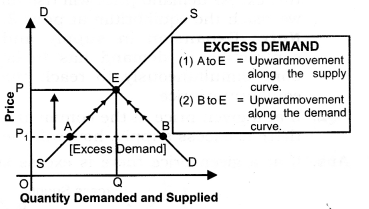In the given diagram the excess demand of AB at price P , creates a competition among the buyers, which will increase the price from P, to P. It can be explained in the following two cases:
Case I: Upward movement along the supply curve (Expansion in Supply) ,
Due to excess demand of AB, competition among the buyers, will rise the price from Pt to P. As we know positive relationship exist between price and quantity supplied- So, the rise in price from P1 to P, will rise the supply from A to E.
Case II: Upward movement along the demand curve (Contraction in Demand)
Due to excess demand of AB, the price rises. As we know Inverse relationship exists between price and quantity demanded. So, due to rise in price from P to P the quantity demanded falls from B to E.
It can also be explained with the help of the schedule that follows: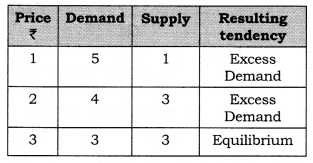In the above schedule, at price 1 there is an excess demand. Due to this excess demand price will rise till we reach the equilibrium at price 3. Note: Expansion in supply and contraction in demand has to be done simultaneously to reach the equilibrium price.

Question 2. If at a given price of the commodity there is excess supply, how will the equilibrium price be reached? Explain with the help of a diagram.
Or [CBSE 2004] How will equilibrium price be reached when there is excess supply? Explain with a diagram.
Or [CBSE 04, 06C, 07C]
Explain the series of changes that will take place if market price is higher than equilibrium price.
Or [CBSE 2011C alternative]
At a given price of a commodity there is excess supply. Is it an equilibrium price? If not, how will the equilibrium price be reached? (use diagram)
Or [CBSE 2006] Suppose price of a good is higher than equilibrium price. Explain changes that will establish equilibrium supply.
[CBSE 09]

Answer: If at a given price there is excess supply as shown in the given figure.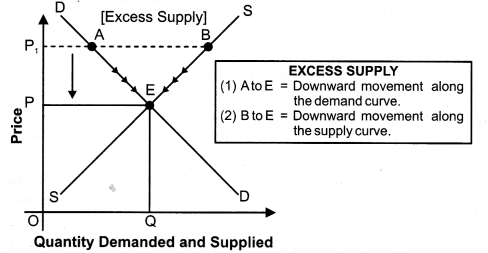In the given figure, the excess supply of AB at price P1 will create a competition among the producers, which will reduce the price from Pj to P. It can be explained in the following cases:

1. Downward movement along the supply curve (Contraction in supply): Due to excess supply of AB, the competition among the producers will reduce the price. As we know, positive relationship exists between price and quantity supplied. So fall in price P1 to P leads to fall in supply from B to E.
2.  Downward movement along the demand curve (Expansion in demand): Due to excess supply of AB, the competition among the producers will reduce the price. As we know inverse relationship exits between price and quantity demanded. So, fall in price from P1 to P, leads to rise in demand from A to E. It can be explained with the help of the following schedule.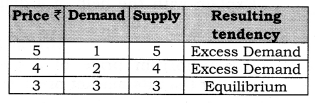In the above schedule at price 5, there is excess supply. This excess supply, leads to fall in price till we reach the equilibrium at price 3. Note: Contraction in supply and expansion in demand have to be done simultaneously to reach the equilibrium.

Question 3. Market for a good is in equilibrium. There is increase in demand for goods. Explain the chain of effects of this change. Use diagram.
Or [CBSE 2011] How does an increase in demand of a commodity affect its equilibrium price and equilibrium quantity? Explain with the help of a diagram.
Or ‘ [CBSE 2005]
How will equilibrium price and quantity be affected when there is rightward shift of demand curve? [CBSE 2004, 07C; AI 05]

Answer:As given in the examination problem that market for a good is in equilibrium. So, we assume that initial price is OP as shown in the figure.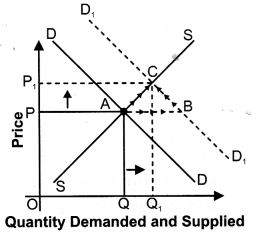In the given figure price is on vertical axis and quantity demanded and supplied are on horizontal axis. But due to increase in demand, demand curve shifts rightward from DD to D1D1 With new demand curve D1D1, there is excess demand at initial price OP because at price OP, demand is PB and supply is PA, so there is excess demand of AB at price OP.
Due to this excess demand, competition among the consumer will rise the price. With the rise in price, there is upward movement along the demand curve (contraction in demand) from B to C and similarly, there is upward movement along the supply curve (expansion in supply) from A to C . So, finally equilibrium price rises from OP to OP1; and equilibrium quantity also rises from OQ to OQ1 Conclusion
Due to increase in demand,

1. Equilibrium price rises from OP to OP1
2. Equilibrium quantity also rises from OQ to OQ1

Question 4. Market for a good is in equilibrium. There is decrease in demand for this good. Explain the chain of effects of this change. Use diagram.
Or
How will equilibrium price and quantity be affected when there is decrease in demand? Explain with diagram. [CBSE 04C, 06C, 09]
Or
How will equilibrium price and quantity be affected when there is leftward shift of demand curve?
Or [AI 2004]
Explain the chain effects on demand, supply and price caused by leftward shift of demand curve.
Or [CBSE 2005 C] Market for a good is in equilibrium. The demand for the good ‘decreases’. Explain the chain of effects of this change. [CBSE 2015 Set(2)]

Answer:  As given in the examination problem that market for a good is in equilibrium. So, we assume that initial price is OP as shown in given figure.
In the given figure price is on vertical axis and quantity demanded and supplied are on horizontal axis. But due to decrease in demand, the demand curve shifts leftward from DD to D1D1. With new demand curve D1D1, there is excess supply at initial price OP because at price OP demand is PB and supply is PA so there is excess supply of AB at price OP.
Due to this excess supply, competition among the producer will make the price fall. Due to fall in price there is downward movement along the demand curve (Expansion in demand) from B to C and similarly there is downward movement along the supply curve (contraction in supply) from A to C. So, finally, the equilibrium price falls from OP to OP1 and equilibrium quantity also falls from OQ to OQ1.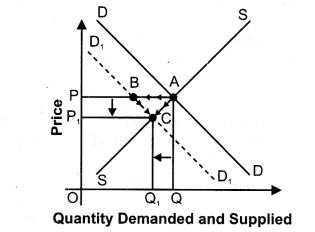Conclusion
Due to decrease in demand,

1. Equilibrium price falls from OP to OP1
2. Equilibrium quantity also falls from OQ to OQ1

Question 5. Market for a good is in equilibrium. There is increase in supply for this goods. Explain the = chain of effects of this change. Use diagram.
[AI2011]
Or
How will equilibrium price and quantity be affected when there is increase in supply?
Or [AI 2005]
Explain the chain effect of increase in supply of a good on its price, supply and demand. Use diagram. [CBSE 05]
Or
How does an increase in supply of a commodity affect its equilibrium price and equilibrium quantity? Explain with the help of a diagram.
[CBSE 2005, OS] Or
Market for a good is in equilibrium. Supply of the good ‘increases’. Explain the chain of effects of this change. [CBSE 2015, AI 2015]

Answer:  As given in the examination problem that market for a good is in equilibrium. So, we assume that initial price is OP as shown in given figure.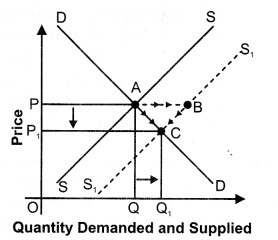In the given figure price is on vertical axis and quantity demanded and supplied is on horizontal axis. But due to increase in supply the supply curve shifts rightward from SS to S1S1. With new supply curve S1S1( there is excess supply at initial price OP because at price OP, supply is PB and demand is PA, so there is excess supply of AB at price OP. Due to this excess supply competition among the producer will make the price fall. Due to this fall in price there is downward movement along the supply curve (Contraction in supply) from B to C and similarly, there is downward movement along the demand curve (Expansion in demand) from A to C. So, finally, equilibrium price falls from OP to OP1 and equilibrium quantity rises from OQ to OQ1.
Conclusion
Due to increase in supply,

1. Equilibrium price falls from OP to OP1
2. Equilibrium quantity rises from OQ to OQ1.

Question 6. Market for a good is in equilibrium. There is decrease in supply for this good. Explain the chain of effects of this change. Use diagram.
[AI 2011] Or
Explain the chain effects of decrease in supply of a good on its price, supply and demand. [CBSE 2005C]

Answer: As given in the examination problem that market for a good is in equilibrium. So,-we assume that initial price is OP as shown in the given figure.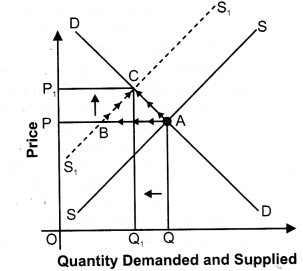In the given figure price is on vertical axis and quantity demanded and supplied is on horizontal axis. But due to decrease in supply the supply curve shifts leftward from SS to S1S1. With new supply curve S1S1, there is excess demand at initial price OP because at price OP, supply is PB and demand is PA, so there is excess demand of AB at price OP.
Due to this excess demand competition among the consumer will rise the price. Due to this rise in price there is upward movement along the supply curve (Expansion in supply) from B to C and similarly, there is upward movement along the demand curve (Contraction in demand) from A to C. So, finally, equilibrium price rises from OP to OP1 and equilibrium quantity falls from OQ to OQ1 Conclusion
Due to decrease in supply,

1.  Equilibrium price rises from OP to OP1
2.  Equilibrium quantity falls from OQ to OQ1.

Question 7. How is the equilibrium price and equilibrium quantity of a normal commodity affected by an increase in the income of its buyers? Explain with the help of a diagram.
Or [CBSE 2006]
Explain the effect of increase in income of buyers of a ‘normal’ commodity on its equilibrium price. [CBSE 2010]

Answer: As, we know normal goods are those whose quantity demanded varies positively with the change in income. As given in the examination problem if income of a consumer rises and goods consumed is normal goods equilibrium price and equilibrium quantity both rise. It can be shown with the help of the given figure.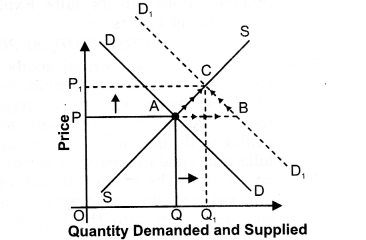In the given figure, price of normal goods is measured on vertical axis and quantity demanded and supplied are measured on horizontal axis. Initially, the equilibrium price is OP and equilibrium quantity is OQ.
But as given in the question when income of a consumer rises the demand of normal goods increases shifting the demand curve to the ‘ right from DD to D1D1.
With new demand curve D1D1, there is excess demand at initial price OP because at price OP demand is PB and supply is PA; so there is excess demand of AB at price OP.
Due to this excess demand, competition among the consumer will raise the price. With the rise i« price there is upward movement along the demand curve (contraction in demand) from B to C and similarly, there is upward movement along the supply curve (expansion in supply) from A to C . So, finally, equilibrium price rises from OP to OP1; and equilibrium quantity also rises from OQ to OQ1.
Conclusion
Due to increase in income of a buyer for normal goods,

1. Equilibrium price rises from OP to OP1
2. Equilibrium quantity also rises from OQ to OQ1

Question  8. How does the equilibrium price of a ‘normal’ commodity change when income of its buyers fall? Explain the chain of effects. [CBSE 2010, AI 2010]
Answer:  As we know that normal goods are those whose quantity demanded varies positively with the change in income. As given in the examination problem if income of a consumer falls and goods consumed is normal goods, then both equilibrium price and the equilibrium quantity fall. It can be shown with the help of the given figure.
In the given figure price of normal goods is measured on vertical axis and quantity demanded and supplied is measured on horizontal axis. Initially, the equilibrium price is OP and equilibrium quantity is OQ.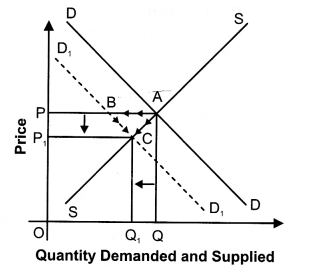But as given in the examination problem when income of a consumer falls the demand of normal goods also falls shifting the demand curve to the left from DD to D1D1.
With new demand curve D1D1 there is excess supply at initial price OP because at price OP demand is PB and supply is PA; so there is excess supply of AB at price OP.
Due to this excess supply competition among the producer will fall the price. Due to fall in price there is downward movement along the demand curve (Expansion in demand) from B to C and similarly, there is downward movement along the supply curve (Contraction in supply) from A to C. So, finally, the equilibrium price falls from OP to OP1 and equilibrium quantity also falls from OQ to OQ1 Conclusion
Due to decrease in income of a buyer for normal goods,
(1) Equilibrium price falls from OP to OP1
(2) Equilibrium quantity also falls from OQ to OQ1

Question 9. How will an increase in the income of buyers of an ‘inferior goods’, affect its equilibrium price and equilibrium quantity? Explain with the help of a diagram. [CBSE 2006]
Answer: As we know inferior goods are those whose quantity demanded varies inversely with the change in income. As given in the examination problem if income of a consumer increases and good consumed is inferior good, equilibrium price and equilibrium quantity both fall. It can be shown with the help of the following figure. In the given figure price of inferior goods is measured on vertical axis and quantity demanded and supplied is measured on horizontal axis. Initially, the equilibrium price is OP and equilibrium quantity is OQ.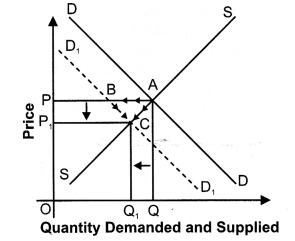But as given in the examination problem when income of a consumer increases, the demand of inferior goods also falls shifting the demand curve to the left from DD to D1D1 With new demand curve D1D1, there is excess supply at initial price OP because at price OP demand is PB and supply is PA; so, there is excess supply of AB at price OP.
Due to this excess supply, competition among the producer the price fall. Due to fall in price, there is downward movement along the demand curve (Expansion in demand) from B to C and similarly, there is downward movement along the supply curve (Contraction in supply) from A to C. So, finally, the equilibrium price falls from OP to OP1, and equilibrium quantity also falls from OQ to OQ1 Conclusion
Due to increase in income of buyer for inferior goods,

1. Equilibrium price falls from OP to OP1
2. Equilibrium quantity also falls from OQ to OQ1

Question 10. How will a fall in price of tea affect the equilibrium price of coffee? Explain the chain of effects. [CBSE 201 IQ]
Answer:  Due to fall in price of tea the demand curve for coffee shifts leftward as shown in the given figure.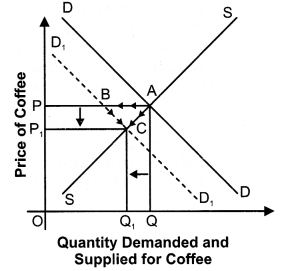In the given diagram price of coffee is on vertical axis and quantity demanded and supplied is on horizontal axis. Initially, the equilibrium price is OP and equilibrium quantity is OQ.
But due to fall in price of tea the demand curve of coffee shifts leftward from DD TO D1D1.
With new demand curve D1D1 there is excess supply at initial price OP because at price OP demand is PB and supply is PA; so, there is excess supply of AB at price OP.
Due to this excess supply competition among the producer will make the price fall. Due to fall in price, there is a downward movement along the demand curve (Expansion in demand) from B to C, and similarly there is a downward movement along the supply curve (Contraction in supply) from A to C. So, finally, the equilibrium price falls from OP to OP1, and equilibrium quantity also falls from OQ to OQ1 So, due to fall in price of Tea,

1. Equilibrium price of coffee falls from OP to OP1, and
2. Equilibrium quantity of coffee also falls from OQ to OQ1.

Question 11. What will be the effect on equilibrium price and equilibrium quantity, when:

1. number of firms increases and
2.  price of inputs increases.

1. Number of firms increases:
When number of firms increase keeping other factors constant, total supply, in the market, also increases due to more producers producing the commodity. It shifts the supply curve towards right. Since an increase in number of firms does not have any impact on demand the demand curve remains unchanged. It can be shown with the help of given diagram.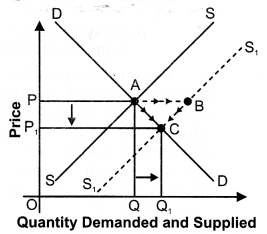The supply curve shifts rightward from SS to S1S1 With new supply curve S1S1 there is excess supply at initial price OP because at price OP supply is PB and demand is PA; so there is excess supply of AB at price OP.
Due to this excess supply, competition among the producer will fall the price. Due to this fall in price there is downward movement along the supply curve (Contraction in supply) from B to C and similarly there is downward , movement along the demand curve (Expansion in demand) from A to C. So, finally, equilibrium price falls from OP to OP1, and equilibrium quantity rises from OQ to OQ1
Conclusion
So, due to increase in number of firms,
(a) Equilibrium price falls from OP to OP1
(b) Equilibrium quantity rises from OQ to OQ1
2. Price of inputs increases: When price of inputs increases, assuming no change in other factors, then the cost of production rises. As a result, supply decreases due to fall in the profitability level. It shifts the supply curve towards left.
Since an increase in the price of inputs does not have any impact on demand, the demand curve remains unchanged. It can be shown with the help of given diagram.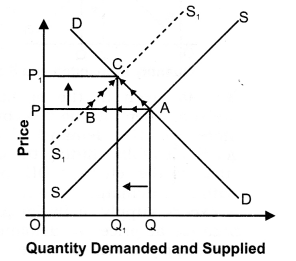In the given figure price is on vertical axis and quantity demanded and supplied is on horizontal axis.
The supply curve shifts leftward from SS to S1S1 With new supply curve S1S1, there is excess demand at initial price OP because at price OP, supply is PB and demand is PA, so there is excess demand of AB at price OP.
Due to this excess demand, competition among the consumer will rise the price. Due to this rise in price, there is upward movement along the supply curve (Expansion in supply) from B to C and similarly, there is upward movement along the demand curve (Contraction in
demand) from A to C. So, finally, equilibrium price rises from OP to OP, and equilibrium quantity falls from OQ to OQ1

Conclusion
Due to increase in input price,

1. Equilibrium price rises from OP to OP1.
2. Equilibrium quantity also falls from OQ to OQ1.

Question 12. What would be an effect on equilibrium price and quantity when demand and supply both increase at the same rate? [CBSE 08, 08C] Or
Explain with the help of a diagram a situation when demand and supply curves shift to the right but equilibrium price remains the same.
[AI 2007] Or
Market for a good is in equilibrium. What is the effect on equilibrium price and quantity if both the market demand and the market supply of the goods increase in the same proportion? Use diagram. [CBSE 2008]

Answer: When demand and supply both increase at the same rate, equilibrium price remains constant and equilibrium quantity rises. It can be shown with the help of the following diagram.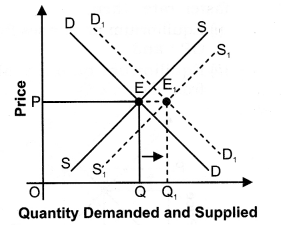In the above diagram price is measured on vertical axis and quantity demanded and supplied is measured on horizontal axis. Initially, the equilibrium price is OP and equilibrium quantity is OQ.
But as given in the examination problem, “demand and supply both increase at the same rate”, then,

1. Equilibrium price remains constant at OP and
2. Equilibrium quantity rises from OQ to OQ1

Question 13. What would be an effect on equilibrium price and equilibrium quantity if demand and supply both fall at the same rate?
Or
Market for a good is in equilibrium. There is simultaneous “decrease” both in demand and supply but there is no change in market price. Explain with the help of a schedule how is it possible. [AI 2012]

Answer: When demand and supply both decrease at the same rate, equilibrium price remains constant and equilibrium quantity falls. It can be shown with the help of the following diagram.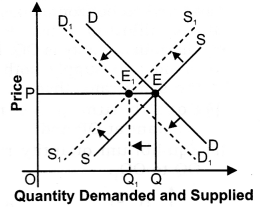In the given diagram price is measured on vertical axis and quantity demanded and supplied is measured on horizontal axis. Initially, the equilibrium price is OP and equilibrium quantity is OQ.
But as given in the examination problem, “demand and supply both decrease at the same rate”, then,

1. Equilibrium price remains constant at OP and
2. Equilibrium quantity falls from OQ to OQ1.

Question 14. What would be an effect on equilibrium price and quantity when demand and supply both shifts rightward?
Or
What would be an effect on equilibrium price and quantity when there is simultaneous increase in demand and supply? [AI 2008] Or
“If the demand and supply of a commodity both increase, the equilibrium price may not change, may increase, may decrease.” Explain using diagrams.
Or [CBSE Sample Paper 2003]
Market for a good is in equilibrium. There is simultaneous “increase” both in demand and supply of the good. Explain its effect on market price. [CBSE 2012]

Case I: When demand and supply both increase at the same rate In the given diagram price is measured on vertical axis and quantity demanded and supplied is measured on horizontal axis. Initially, the equilibrium price is OP and equilibrium quantity is OQ. But when “demand and supply both increase at the same rate” then,
(i) Equilibrium price remains constant at OP and
(ii) Equilibrium quantity rises from OQ to OQ1.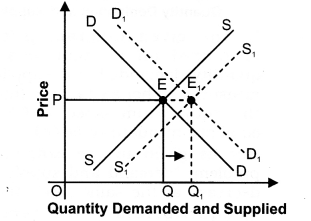Case II: When demand increases, supply also increases but at a much faster rate
In the given diagram price is measured on vertical axis and quantity demanded and supplied is measured on horizontal axis. Initially, the equilibrium price is OP and equilibrium quantity is OQ. But when “demand increases and supply also increases but at a much faster rate”, then,
(i) Equilibrium price falls from OP to OP1 and
(ii) Equilibrium quantity rises from OQ to OQ1.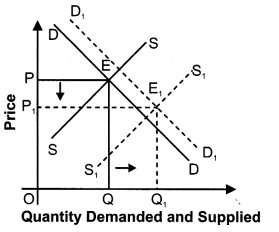Case III: When supply increases, demand also increases but at a much faster rate
In the given diagram price is measured on vertical axis and quantity demanded and supplied is measured on horizontal axis. Initially, the equilibrium price is OP and equilibrium quantity is OQ. But when “supply increases and demand also increases but at a much faster rate” then, ,
(i) Equilibrium price rises from OP to OP1; and
(ii)Equilibrium quantity also rises from OQ to OQ1.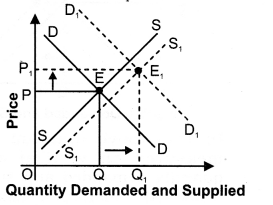VI. Higher Order Thinking Skills
Question 1. What happens to equilibrium price of a commodity if there is an ‘increase’ in its demand and ‘decrease’ in its supply? [ 1 Mark]

Question 2. What happens to equilibrium price of a commodity if there is an ‘decrease’ in its demand and ‘increase’ in its supply? [ I Mark]

Question 3. “Demand and supply are like two blades of a pair of scissors”. Comment. [3 Marks]
Answer: The given statement is correct. Both the blades of a pair of scissors are equally important to cut a piece of cloth. Similarly, both demand and supply are needed for determining price in the market. There is no use for demand for a product if there is no supply for the product and supply is not needed if there is no demand for the product. One of the two may play more active role in price determination in the short run. But, both are needed to determine the price in the long run.

Question 4. Mention the various cases in which equilibrium price remains same.
[3 Marks]

Answer: The equilibrium price remains same in the following cases:

1. When increase in demand is equal to increase in supply.
2. When decrease in demand is equal to decrease in supply.
3. When demand increases and supply is perfectly elastic.
4. When demand decreases and supply is perfectly elastic.
5.  When supply increases and demand is perfectly elastic.
6. When supply decreases and demand is perfectly elastic.

VII. Value Based Questions
Question 1. Why has power crisis increased in India? [ 1 Mark]
Answer: Because its demand is greater than supply.
Value: Awareness about efficient use of power.

Question 2. The market price of sugar rises when its demand increases. How can the supply of sugar be changed so that price of sugar remains constant? [1 Mark]
Answer: The price of sugar remains constant when demand and supply increase in the same proportion.
Value: Problem solving.

Question 3. Explain the effects on the market equillibrium by imposing ban on the sale of GUTKHA in Delhi. [1 Mark]
Answer: Due to the ban on the sale of GUTKHA in Delhi, the supply of GUTKHA will be reduced and the firms producing GUTKHA will shift their factors of production in the production of other related goods. Consumer demand of GUTKHA will reduce and hence in this way both the demand and supply of GUTKHA will reduce.
Value: Social health conciousness.

Question 4. Consumers often suffer because of their ignorance about the market situations and pay higher price than the equilibrium price. How can this be avoided? ]1 Mark]
Answer: Consumers should be vigilant and aware about the market situation,i.e., they should know the prevailing market prices. In +his way they will not suffer because of being ignorant. Value: Awareness about Market situation.

Question 5. Business Process Outsourcing (BPO) is bringing in attractive jobs to the educated urban youths in India. Though highly remunerative, these jobs involve long and inconvenient working hours. What is the impact of BPO on the supply of labour? [1 Mark]
Answer: With the increase in demand higher wages are being paid to BPO workers. In response the supply of labour has been increasing.
Value: Analytic

Question 6. Equilibrium price of an essential medicine is too high. Explain what possible steps can be taken to bring down the equilibrium price but only through the market forces. Also explain the series of changes that will occur in the market.
[AI 2013][6 Marks]

Answer:  Following are the possible steps that can be taken to bring down the equilibrium price:

1. The government should provide subsidy on the production of such medicines. _ .
2. The government should cut down or abolish all the taxes on such medicine.
3. The government should offer various facilities to industrialists to motivate them for the production of such medicine. These facilities will reduce the cost of inputs used in the production of medicines. The effect of above steps will be that supply of such medicines will increase and as the result of, the equilibrium price of medicines will reduce as shown in the given figure.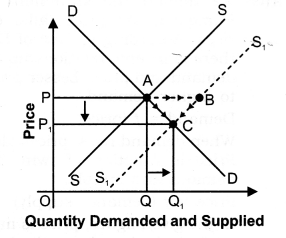In the given figure price is on vertical axis and quantity demanded and supplied is on horizontal axis. But due to increase in supply the supply curve shifts rightward from SS to S1S1. With new supply curve S1S1, there is excess supply at initial price OP because at price OP, supply is PB and demand is PA, so there is excess supply of AB at price OP. Due to this excess supply competition among the producer will make the price fall. Due to this fall in price there is downward movement along the supply curve (Contraction in supply) from B to C and similarly, there is downward movement along the demand curve (Expansion in demand) from A to C. So, finally, equilibrium price falls from OP to OP1 and equilibrium quantity rises from OQ to OQ1

Conclusion
Due to increase in supply,
(i) Equilibrium price falls from OP to OP1
(ii) Equilibrium quantity rises from OQ to OQ1
Value: Analytic

Question 7. The following headline appeared in the Hindustan Times on 2 August 2014:
“Crop damaged in Himachal sent tomato prices roaring in Delhi.”
Use a diagram and economic theory to analyse the statement.
[CBSE Sample Paper 2014][6 Marks]

Answer: When the tomato crop was damaged in Himachal the supply of tomatoes decreases. This means that the supply curve shifts leftward from SS to S1S1.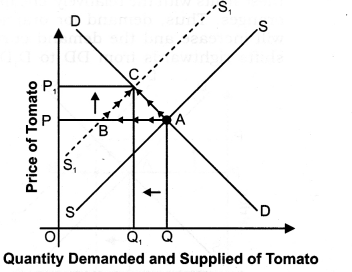With new supply curve S1S1, there is excess demand at initial price OP because at price OP, supply is PB and demand is PA, so there is excess demand of AB at price OP.
Due to this excess demand, competition among the consumer will raise the price of tomato. Due to this rise in price of tomato, there is upward movement along the supply curve (Expansion in supply) from B to C and similarly, there is upward movement along the demand curve (Contraction in demand) from A to C. So, finally, equilibrium price of tomato rises from OP to OP1 and equilibrium quantity of tomato falls from OQ to OQ1 Conclusion:
This explains how prices in Delhi rose when the tomato crop got damaged in Himachal.
Value: Analytic

Question 8. On 19 December 2013, the following news item was printed in the Economic Times:Households in Southern India prefer to eat oranges for breakfast as banana plantations in Kerala have been destroyed and price of apples and grapes have also risen.
Use a diagram and economic theory to analyse the impact of the rise in price of apples and grapes on the market of oranges. [CBSE Sample Paper 2014][6 Marks]

Answer: When the price of apples and grapes rises, consumers will substitute, with these fruits with the relatively cheaper oranges. Thus, demand for oranges will increase and the demand curve shifts rightwards from DD to D1D1.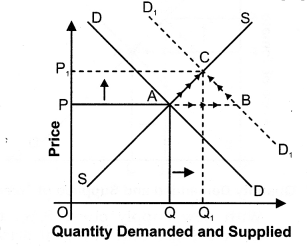With new demand curve D1D1, there is excess demand at initial price OP because at price OP demand is PB and supply is PA; so there is excess demand of AB at price OP.
Due to this excess demand, competition among the consumer will raise the price. With the rise in price there is upward movement along the demand curve (contraction in demand) from B to C and similarly, there is upward movement along the supply curve(expansion in supply) from A to C . So, finally, equilibrium price rises from OP to OP1, and equilibrium quantity also rises from OQ to OQ1
Note: Therefore, the equilibrium price of oranges increases and the equilibrium quantity also increases when the price of apples and grapes rises in Southern India.
Value: Analytic

VIII. Application Based Questions
Question 1. Mrs Ramgopal says that economists say inconsistent things: as price falls, demand rises but as demand rises, prices rises. Defend or refute. [3-4 Marks]
Answer: We defend the statement of Mrs Ramgopal. As price falls, demand rises. According to Law of Demand, there is inverse relationship between demand and price. Leaser price leads to higher demand.
Demand = f (price)
When demand rises, prices also rises. Price is function of twin forces of demand and supply.
Price = f (demand, supply)
In the given figure, price is measured on vertical axis and quantity demanded and supplied is measured on horizontal axis. Initially, the equilibrium price is OP and equilibrium quantity is OQ. But due increase in demand, the demand curve shifts rightward from DD to D1D1.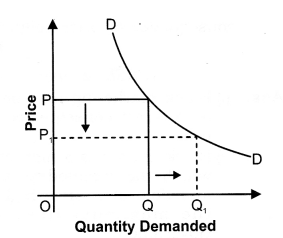With new demand curve D1D1, there is excess demand at initial price OP because at price OP, demand is PB and supply is PA, so there is excess demand of AB at price OP.
Due to this excess demand, competition among the consumer will raise the price. With the rise in price, there is upward movement along the demand curve (contraction in demand) from B to C and similarly, there is upward movement along the supply curve (expansion in supply) from A to C . So, finally equilibrium price rises from OP to OP1 So, demand rises, price rises.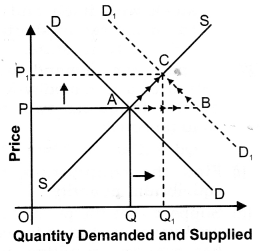Question 2. “An increase in the demand for notebooks raises the quantity of notebooks demanded, but not the quantity supplied.” Is this statement true or false? Explain. [3-4 Marks]
Answer: The statement that “an increase in the demand for notebooks raises the quantity of notebooks demanded, but not the quantity supplied,” in general, is false. As given figure shows, the increase in demand for notebooks results in an increased quantity supplied.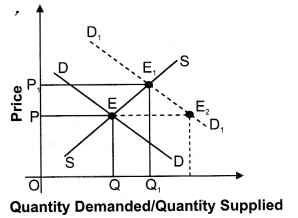Question 3. China is a big manufacturer of telephone instruments. It has recently become a member of WTO, which means that it can sell its product in other member countries like India. Suppose that it does export a large number of telephone instruments to India. [ 6 Marks]

1. How will it affect the price and quantity sold of telephone instruments in India?
2. Suppose that the demand for telephone instruments is relatively elastic. How will it affect India’s total expenditure on telephone instruments?

1. The supply of telephone instruments in India will increase. An increase in supply of telephone instruments will result in a . rightward shift of the supply curve from SS to S1S1 as shown below: The supply curve shifts rightward from SS to S1S1. With new supply curve S1S1 there is excess supply at initial price OP because at price OP supply is PB and demand is PA; so there is excess supply of AB at price OP.
2. Due to this excess supply, competition among the producer will fall the price. Due to this fall in price there is downward movement along the supply curve (Contraction in supply) from B to C and similarly there is downward movement along the demand curve (Expansion in demand) from A to C. So, finally, equilibrium price falls from OP to OP1, and equilibrium quantity rises from OQ to OQ1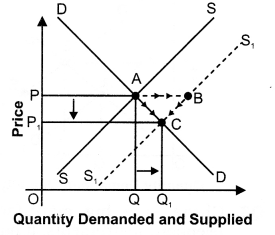Conclusion
Due to increase in number of firms,
(a) Equilibrium price of telephones falls from OP to OP1
(b) Equilibrium quantity of telephones rises from OQ to OQ1.
For a product with relatively elastic demand a fall in the price of a commodity results in a relatively larger expenditure by the consumers. Thus, the total expenditure on telephones will increase.

Question 4. Given below are four statements.Indicate for each whether it reflects an increase or decrease in demand;quantity demanded; supply; quantity supplied. [3-4 Marks]

1. Air Deccan reduces its average plane fare by 30% in order to attract more passengers.
2. The government grants export subsidy to producers of oranges in Nagpur to increase the sale of oranges abroad.
3. Wheat farmers decide to withhold wheat as the market price is low.
4. OPEC decides to increase the international oil price.

1. Increase in quantity demanded
2. Increase in supply.
3. Decrease in supply.
4. Decrease in quantity demanded.

Question 5. Explain “black marketing’ as a direct [3-4 Marks] [CBSE Sample Paper 2016]

1. Black marketing may be termed as a direct consequence of price ceiling.
2. Black market is a market under which the commodity is bought and Sold at a price higher than the maximum fixed by the Government.
3. It arises due to presence of consumers who may be willing to pay higher price for the commodity than to go without it.

Question 6. Explain the concept of “buffer stock’ as a tool of price floor. [3-4 Marks]
[CBSE Sample Paper 2016]